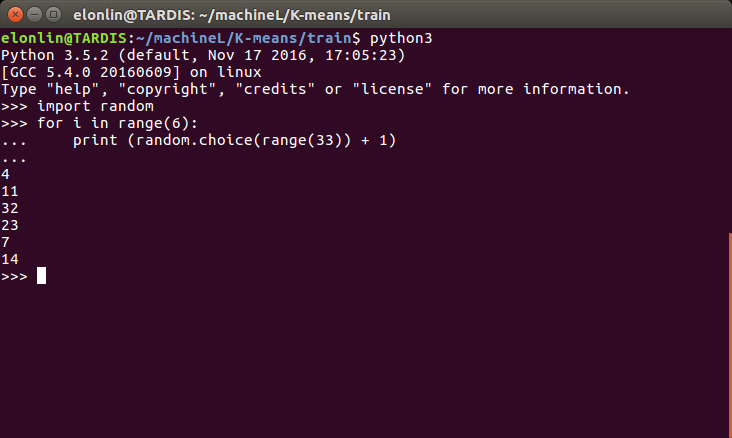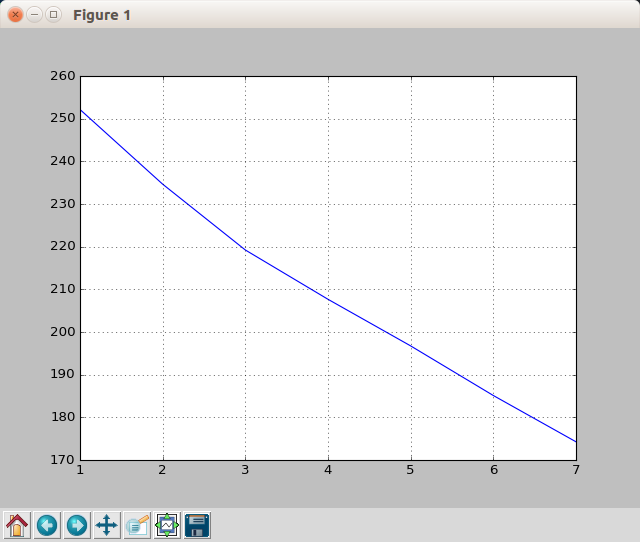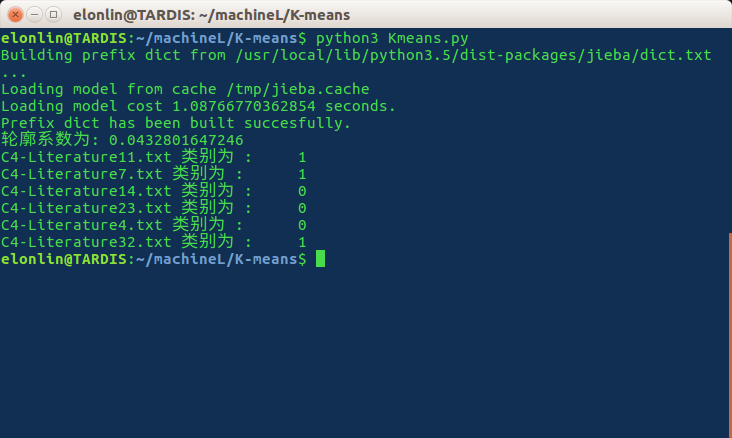@ArrowLLL 2017-04-18T04:28:57.000000Z 字数 5881 阅读 3623

# K-Means 文本聚类

python Kmeans 机器学习

## 参考

• jieba分词组件组件介绍
• Introduction to K-means Clustering
• 《Python机器学习及实践:从零开始通往Kaggle竞赛之路》中 K-means聚类部分
• Matplotlib 教程
• 轮廓系数的两个参考 : Silhouette (clustering)轮廓系数-百度百科

## 准备

• python版本 : 3.5.2

• 操作系统 : ubuntu 16.04
• 用到的python库 :
• jieba -- 用于中文分词， 可以通过sudo pip3 install jieba3k 直接安装
• numpy, 科学计算库，通过sudo pip3 install numpy 安装
• scikit-learn, 数据挖掘库, 可以通过 sudo pip3 install scikit-learn 安装
• pylab, 绘图工具库。可以通过sudo pip3 install matplotlib 安装
• 33 份新闻文稿。以 $8 : 2$ 的比例挑选训练集与测试集。即随机从33 份新闻文稿中挑选6份作为测试集。

## 文档树

.
├── findK.py
├── Kmeans.py
├── pycache
│      └── findK.cpython-35.pyc
├── test
│      ├── C4-Literature11.txt
│      ├── C4-Literature14.txt
│      ├── C4-Literature23.txt
│      ├── C4-Literature32.txt
│      ├── C4-Literature4.txt
│      └── C4-Literature7.txt
├── train
│      ├── C4-Literature1.txt
│      ├── ...
│      └── C4-Literature32.txt
└── tree.txt

3 directories, 37 files

• findK.py 是从训练集中寻找K-means算法中合适的K值的python程序，后文中会介绍这种方法;
• Kmeans.py 是K-means算法的程序,包括训练与测试;
• pycache/ 文件夹是 K-means.py 调用 findK.py 中的函数生成的文件夹，其下的 findK.cpython-35.pyc 也是调用后生成的相应的.pyc文件, 方便下一次装载;
• test/ 文件夹下是测试集;
• train/ 文件夹下是训练集;
• tree.txt 是通过 ~\$ tree > tree.txt 命令生成的文档树文件，就是上面引用中的内容，此处为了方便展示改变了一下树的姿势，将 train/ 文件夹下的文本用省略号代替了一部分;## 模型框架

### 提取特征

• getTags() 函数遍历 train/ 文件夹下的文本，使用 jieba.analyse包里的函数 extract_tags() 函数提取文本的关键词，对每篇文本提取的关键字个数topK为10个。然后将其存入文本关键词列表 wordlist 和关键词位置字典 wordDic 并返回;
• getMatrix(wordDic, wordlist) 显然其参数是getTags()的两个返回列表。这个函数的作用是将每个文本的关键字列表转化为一个01矩阵，每一行代表一个文本，每一列是一个关键词，如果第i个文本含有第j个关键词，则有 wordMartix[i, j] = 1 , 如果第i个文本不含有第k个关键词，则有 wordMatrix[i, k] = 0 , 对所有文本操作完毕后返回这个01矩阵。

### 找k值### K-Means聚类

kmeans聚类使用的是sk-learn自带的KMeans类KMeans()。参数n_clusters表示聚类中心的个数。中使用到的属性和方法如下：

• KMeans.fit(X) 表示运用Kmeans算法计算X矩阵;
• KMeans.cluster_centers_表示聚类中心点;
• KMeans.lables_ 表示各个聚类样本的聚类结果标签;

## 代码及其相应注释

### findK.py

#!usr/bin/python3#coding: utf-8import osimport jiebaimport jieba.analyseimport numpy as npfrom sklearn.cluster import KMeansfrom pylab import *# 提取训练集中文本的关键字def getTags() :    path = './train/'    wordDic, wordslist = {}, []    # os.listdir() 提取相应路径下的文件名    for fileName in os.listdir(path) :        # 以gbk格式打开文本        with open(path + fileName, encoding = 'gbk') as f :            # extract_tags() 函数提取关键字            text = ' '.join(jieba.analyse.extract_tags(f.read(), topK = 10))            words = text.split()            # 将当前文本关键字放入总的关键字列表中            wordslist.append(words)            # 将当前文本关键字放入关键字字典中            for word in words :                wordDic[word] = 0    # 遍历官架子字典，为每一个关键字取定一个字段值，即列号存入字典    for (word, seqNo) in zip(wordDic, range(len(wordDic))) :        wordDic[word] = seqNo    return wordDic, wordslistdef getMatrix(wordDic, wordslist) :    # 取定第一维为文本数，第二维为关键字数量的零矩阵    wordMatrix = np.zeros([len(wordslist), len(wordDic)])    # 第i个文本包含第j个词，则wordMatrix[i, j] = 1,构造01矩阵    for (i, words) in zip(range(len(wordslist)), wordslist) :        for word in words :            wordMatrix[i, wordDic[word]] = 1    # 返回01矩阵    return wordMatrixif __name__ == '__main__' :    # 提取文本关键字列表与关键词字典    wordDic, wordslist = getTags()    # 构造关键词01矩阵    wordMatrix = getMatrix(wordDic, wordslist)    # n 表示聚类数量K的封顶值    n, distance = 8, []    for i in range(1, n) :        # 初始化最小距离为 -1        minDis = -1        # 跑10次Kmeans以保证取得的是全局最优解（10可以更大）        for j in range(10) :            # 调用sklearn的kmeans类            kmeans = KMeans(n_clusters = i).fit(wordMatrix)            # centers 表示每个样本对应的聚类中心点            centers = np.array([kmeans.cluster_centers_[k] for k in kmeans.labels_])            # 计算当前Kmeans结果的距离，取欧式距离但没有开方            dis = ((wordMatrix - centers) ** 2).sum()            # 更新最小值            minDis = dis if minDis < 0 else min(minDis, dis)        # 将相应的k值存入distance列表        distance.append(minDis)    # 绘图    plot(range(1, n), distance)    # 绘制网格    grid()    show()

### Kmeans.py

#!usr/bin/python3#coding: utf-8import osimport numpy as npimport jieba.analyse as jbaysfrom sklearn.cluster import KMeansfrom sklearn.metrics import silhouette_scoreimport findK# 获取测试集的关键词01矩阵，与findK.py 中getMatrix()函数基本相同，不再做过多解释def getTestMatrix(wordDic, matrixshape1) :    path = './test/'    keyWordslist = []    for fileName in os.listdir(path) :        with open(path + fileName, encoding = 'gbk') as f :            text = ' '.join(jbays.extract_tags(f.read(), topK = 10))            words = text.split()            keyWordslist.append(words)    matrixshape0 = len(os.listdir(path))    testMatrix = np.zeros((matrixshape0, matrixshape1))    for (i, keywords) in zip(range(matrixshape0), keyWordslist) :        for keyword in keywords :            if keyword in wordDic :                testMatrix[i, wordDic[keyword]] = 1    return os.listdir(path), testMatrixif __name__ == '__main__' :    # 获得训练集的关键词字典与列表    worDic, wordslist = findK.getTags()    # 获得训练集关键词的01矩阵    minDis, wordMatrix = -1, findK.getMatrix(worDic, wordslist)    # 获得测试集的文本标题与测试集关键词01矩阵    fileName, testMatrix = getTestMatrix(worDic, wordMatrix.shape)    # 奔跑10次KMeans防止取到局部最优解，与findK.py中类似，不过多解释    for j in range(10) :        # 取k值为3        kmeans = KMeans(n_clusters = 3).fit(wordMatrix)        centers = np.array([kmeans.cluster_centers_[k] for k in kmeans.labels_])        dis = ((wordMatrix - centers) ** 2).sum()        if minDis < 0 or dis < minDis :            minDis,testKmeans = dis, kmeans    # 使用轮廓系数评价聚类模型    siCoScore = silhouette_score(wordMatrix, testKmeans.labels_, metric='euclidean')    print ('轮廓系数为:', siCoScore)    # 预测测试集的分类结果    result = testKmeans.predict(testMatrix)    for i in range(result.shape) :        print (fileName[i] + ' 类别为 :\t', result[i])

## 最终结果• 私有
• 公开
• 删除